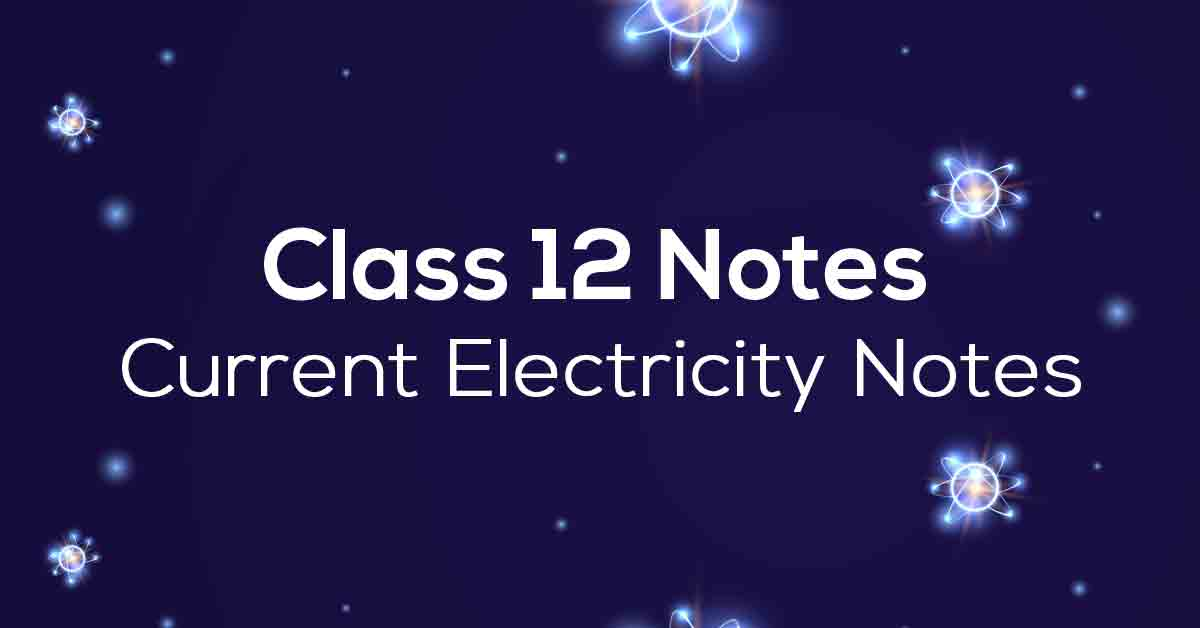Class 12th Physics Current Electricity NCERT Notes CBSE 2023

# Current Electricity Class 12 Notes# Chapter 3 Current Electricity

Currents are an important chapter for NCERT Grade 12 Physics. The notes provided by Vidyakul are essential resources to help students achieve high scores in the Grade 12 Physics Entrance and Examination.

The subject experts have developed this chapter following the latest CBSE 2022 Syllabus guidelines. The notes provided by Vidyakul for Chapter 3 Physics Grade 12 contain all the answers to the NCERT exercise questions and intertextual questions.

### Points to Remember

Below we have provided some of the important points to remember for NCERT Solutions for Physics Chapter 3 to ace your exam:

• A conductor’s current can be measured by its rate of flow of electric charge through its cross-section.

• When the area of the cross-section of the conductor is held normal to the direction of the flow of current, the current density at a point in the conductor equals the ratio of current to the area.

• In solids, all metals conduct electricity well, and free electrons are responsible for this.

• Drift velocity is the average velocity with which free electrons move towards a conductor’s positive end when an external electric field is applied.

• Mobility is the ratio between the electron’s drift velocity and the applied electric field.

• A temperature coefficient of resistivity measures how much resistivity increases per unit increase in temperature when resistivity increases linearly with temperature.

### Topics and Sub-topics

You must know the topics and topics of the NCERT Grade 12 Chapter 3 notes. Accurate knowledge of the topics in the Grade 12 Physics Chapter 3 NCERT notes will help you retain information. The notes given by Vidyakul are of high quality and the detailed explanations make it easy for students to grasp the concepts.

At Vidyakul, we have provided all the necessary information regarding the following:

Click on the topic-wise links provided in the table below to get access to the CBSE Physics Chapter 3 chapter-wise important questions:

 Section Name Topic Name Description 3.1 Introduction 3.2 Electric Current 3.3 Electric Currents in Conductors 3.4 OHM’s Law 3.5 Drift of Electrons and the Origin of Resistivity 3.5.1 Mobility 3.6 Limitations of OHM’s Law 3.7 Resistivity of Various Materials 3.8 Temperature Dependence of Resistivity 3.9 Electrical Energy, Power 3.10 Combination of Resistors- Series and Parallel 3.11 Cells, EMF, Internal Resistance 3.12 Cells in Series and in Parallel 3.13 Kirchhoff’s Rules 3.14 Wheatstone Bridge 3.15 Meter Bridge 3.16 Potentiometer

Download Vidyakul App for more Handwritten notes, PDF's and Free video lectures.

### Few Important Questions

• An electric dipole has the magnitude of its charge as q and its dipole moment is p. It is placed in a uniform electric field E. If its dipole moment is along the direction of the field, then what would be the net force acting on it and its potential energy?

If its dipole moment is along the direction of the field, the net force acting on it is zero and its potential energy is minimum.

• If E is the electric field intensity of an electrostatic field, then the electrostatic energy density is proportional to?

Electrostatic energy density is proportional to E2.

• If a charged body is placed near a neutral conductor, then how it will impact the conductor?

In order to maintain the conductor neutral, an exactly equal amount of opposite charge will appear on the opposite surface of the conductor. Thus attractive forces will dominate and charged body will attract the conductor.

### Practice Questions

1. A spherical conductor has a charge of 4 × 10–8C distributed uniformly on its surface of radius 15 cm. Calculate the electric field just outside the sphere and at a point 15 cm from the center of the sphere.

1. Find out the capacitance if the distance between the plates is reduced by half and the parallel plate capacitor has a capacitance of 20 pF (1pF = 10-12 F) with air between the plates.

2. What is the total capacitance of the combination Three capacitors each of capacitance 20 pF are connected in series.